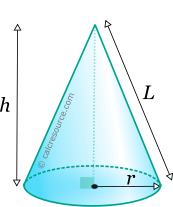Properties of Cone

This tool calculates the basic geometric properties of a cone, with a circular base. Enter the shape dimensions 'r' and 'h' below. The calculated results will have the same units as your input. Please use consistent units for any input.

 r = h =
 Geometric properties: Volume = Surface area = Base area = Lateral surface area = Lateral height L =Definitions

Geometry

The cone is a solid object with a circular base and a side surface that converges to a single point, the apex.

The volume of a cone is given by the formula:

where r the radius of the circular base and h the height of the cone. This formula is derived from the following general expression, that also covers all pyramids, with any polygonal base:

where the base area.

The lateral surface area of the cone can be found using the following formula:

where L the lateral height of the cone, which is easily found as:

Since the area of the circular base is equal to , the total surface area of the cone is: